The SSM Procedure

Example 27.11 Panel Data: Dynamic Panel Model for the Cigar Data

This example shows how you can use the SSM procedure to specify and fit the so-called dynamic panel model, which is commonly used to analyze a panel of time series. Suppose that a panel of time series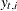follows the model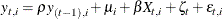where t denotes the time index (for example,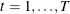); i denotes the panel index (for example,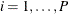);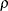is the autoregression coefficient;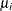denote the panel-specific intercepts;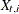are observations on a regression variable with regression coefficient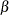(the same for all panels);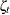are unobserved, random time effects; and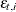are the observation errors. The sequencesandare assumed to be independent, zero-mean Gaussian variables with variances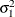and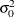, respectively. This is an example of a dynamic panel model that contains one regressor variable. It is easy to formulate this model equation as a state equation with state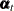of size P—the number of panels. Taking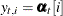, it is easy to see that the statesevolve according to the equation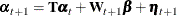where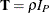(a P-dimensional, diagonal matrix with all its diagonal elements equal to);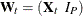is a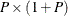-dimensional matrix (in a block form) of state regression variables, where the first block is a column that includes all the valuesthat are associated with a given time index (t) and the second block is a P-dimensional identity matrix;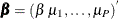is the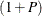-dimensional column vector of regression coefficients; and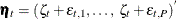is a P-dimensional column vector of all the disturbances that are associated with time index t. Becauseandare independent, the covariance matrix of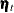—for example,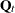—is easy to calculate: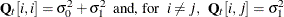. This formulation can be easily extended to multiple regression variables, such asvariables, by appropriately modifying the term that is associated with the state regression variables—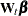: the new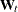matrix becomes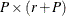-dimensional and the new regression vector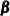becomes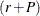-dimensional.

The cross-sectional data, Cigar, that are used in the section Getting Started: SSM Procedure are reused in this example. In order to use the SSM procedure to perform the dynamic panel model–based analysis, the input data set must be reorganized so that it contains the variables that form the-dimensional matrix. For the Cigar data, the number of panels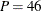(the number of regions considered in the study), and the number of regression variables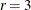. Therefore, the input data set needs to be augmented by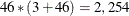variables that constitute the matrix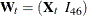—the first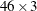-dimensional block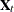contains the values of the three regression variables, lprice, lndi, and lpimin, at a given time index (a particular year in this case). The following DATA steps accomplish this task in two steps. In the first step, the raw data that form the rows of the Cigar data set are read into a temporary data set, Tmp, such that all 6*46 = 276 values that are associated with a given year (values of six variables—year, region, lsales, lprice, lndi, and lpimin for 46 panels in a given year) are read in a single row that consists of 276 columns. In the second step, the final input data set is formed by rearranging Tmp so that it contains the necessary variables in the proper order—year (the time index), region (the panel index), lsales (the response variable), and the variables that form the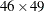-dimensional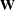matrix (w1, . . ., w2254).

data Tmp;
input u1-u276;
datalines;
63 1 4.54223 3.35341 7.3514 3.26194
63 2 4.82831 3.17388 7.5729 3.21487
63 3 4.63860 3.29584 7.3000 3.25037

... more lines ...


data cigar(keep=year region lsales w1-w2254);
array wmat{46, 49} w1-w2254;
array ivar{46, 6} u1-u276;
set tmp;
year = intnx( 'year', '1jan63'd, u1-63 );
format year year.;
do i=1 to 46;
region = ivar[i, 2];
lsales = ivar[i, 3];
do j=1 to 46;
do k=1 to 49;
wmat[j,k] = 0;
if k = j+3 then wmat[j,k] = 1;
if k=1 then wmat[j,k] = ivar[j, 4];
if k=2 then wmat[j,k] = ivar[j, 5];
if k=3 then wmat[j,k] = ivar[j, 6];
end;
end;
output;
end;
run;


The following statements specify and fit the dynamic panel model:

 proc ssm data=Cigar opt(tech=dbldog maxiter=75);
id year interval=year;
parms rho / lower=-0.9999 upper=0.9999;
parms sigma0 sigma1 / lower=1.e-8;
array RegionArray{46} region1-region46;
do i=1 to 46;
RegionArray[i] = (region=i);
end;
array cov{46,46};
do i=1 to 46;
do j=1 to 46;
if(i=j) then cov[i,j] = sigma0 + sigma1;
else cov[i,j] = sigma1;
end;
end;
state panelState(46) T(I)=(rho) W(g)=(w1-w2254)
cov(g)=(cov) a1(46) checkbreak;
comp dynPanel = (RegionArray)*panelState;
model lsales = dynPanel;
output out=for1 press;
run;


The estimates of the regression coefficients and the regional intercepts, which are all statistically significant, are shown in Output 27.11.1. In particular, the estimated coefficients of lprice, lndi, and lpimin, are –0.26, 0.13, and 0.07, respectively.

Output 27.11.1: Estimates of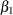,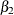,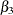and the Regional Intercepts

The SSM Procedure

Estimate of the State Equation Regression Vector
State Element Index Estimate Standard
Error
t Value Pr > |t|
panelState 1 -0.2627 0.0178 -14.79 <.0001
panelState 2 0.1340 0.0130 10.30 <.0001
panelState 3 0.0748 0.0198 3.78 0.0002
panelState 4 0.4265 0.0581 7.35 <.0001
panelState 5 0.3825 0.0605 6.32 <.0001
panelState 6 0.4425 0.0582 7.61 <.0001
panelState 7 0.3471 0.0631 5.50 <.0001
panelState 8 0.3686 0.0635 5.81 <.0001
panelState 9 0.4357 0.0614 7.10 <.0001
panelState 10 0.3753 0.0655 5.73 <.0001
panelState 11 0.4249 0.0606 7.01 <.0001
panelState 12 0.4185 0.0604 6.92 <.0001
panelState 13 0.3824 0.0602 6.35 <.0001
panelState 14 0.3942 0.0644 6.12 <.0001
panelState 15 0.4154 0.0626 6.64 <.0001
panelState 16 0.3961 0.0610 6.49 <.0001
panelState 17 0.3765 0.0618 6.10 <.0001
panelState 18 0.4528 0.0608 7.44 <.0001
panelState 19 0.4316 0.0586 7.36 <.0001
panelState 20 0.4357 0.0601 7.25 <.0001
panelState 21 0.3771 0.0639 5.90 <.0001
panelState 22 0.3939 0.0629 6.26 <.0001
panelState 23 0.4122 0.0621 6.64 <.0001
panelState 24 0.3949 0.0605 6.52 <.0001
panelState 25 0.4386 0.0565 7.77 <.0001
panelState 26 0.4118 0.0627 6.57 <.0001
panelState 27 0.3898 0.0604 6.45 <.0001
panelState 28 0.3818 0.0613 6.23 <.0001
panelState 29 0.4343 0.0632 6.87 <.0001
panelState 30 0.4619 0.0625 7.39 <.0001
panelState 31 0.3730 0.0636 5.86 <.0001
panelState 32 0.3784 0.0589 6.43 <.0001
panelState 33 0.3825 0.0625 6.12 <.0001
panelState 34 0.3784 0.0598 6.32 <.0001
panelState 35 0.4093 0.0628 6.52 <.0001
panelState 36 0.4155 0.0597 6.96 <.0001
panelState 37 0.3960 0.0615 6.44 <.0001
panelState 38 0.4075 0.0602 6.77 <.0001
panelState 39 0.4045 0.0586 6.91 <.0001
panelState 40 0.3918 0.0599 6.55 <.0001
panelState 41 0.4350 0.0608 7.16 <.0001
panelState 42 0.4007 0.0602 6.65 <.0001
panelState 43 0.3196 0.0597 5.36 <.0001
panelState 44 0.4337 0.0609 7.12 <.0001
panelState 45 0.3790 0.0634 5.98 <.0001
panelState 46 0.3767 0.0618 6.10 <.0001
panelState 47 0.4392 0.0597 7.36 <.0001
panelState 48 0.3932 0.0603 6.51 <.0001
panelState 49 0.3938 0.0616 6.40 <.0001

Output 27.11.2 shows the estimates of the autoregression coefficient, the observation error variance, and the variance of the time effect (variance of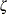).

Output 27.11.2: Estimates of,, andEstimates of Named Parameters
Parameter Estimate Standard
Error
rho 0.831679 0.0124338
sigma0 0.001231 0.0000491
sigma1 0.000213 0.0000662

Finally, you can compare the fit of the dynamic panel model with the fit of the model that is discussed in the section Getting Started: SSM Procedure. Output 27.11.3 shows the likelihood-based information criteria for the dynamic panel model, and Output 27.11.4 shows the same information for the other model.

Output 27.11.3: Likelihood-Based Information Criteria: Dynamic Panel Model

Information Criteria
Statistic Diffuse Likelihood
Based
Profile Likelihood
Based
AIC (lower is better) -4732.722 -4856.398
BIC (lower is better) -4717.247 -4343.874
AICC (lower is better) -4732.704 -4841.250
HQIC (lower is better) -4726.913 -4664.667
CAIC (lower is better) -4714.247 -4245.874

Output 27.11.4: Likelihood-Based Information Criteria: Getting Started Example

Information Criteria
Statistic Diffuse Likelihood
Based
Profile Likelihood
Based
AIC (lower is better) -4488.093 -4145.246
BIC (lower is better) -4477.776 -3637.952
AICC (lower is better) -4488.084 -4130.417
HQIC (lower is better) -4484.220 -3955.472
CAIC (lower is better) -4475.776 -3540.952

Similarly, Output 27.11.5 shows fit criteria based on the delete-one cross validation error for the dynamic panel model, and Output 27.11.6 shows the same information for the other model.

Output 27.11.5: Delete-One Cross Validation Criteria: Dynamic Panel Model

Delete-One Cross Validation Error Criteria
Variable N PRESS Generalized Cross-Validation
lsales 1380 1.115309 5.62798E-7

Output 27.11.6: Delete-One Cross Validation Criteria: Getting Started Example

Delete-One Cross Validation Error Criteria
Variable N PRESS Generalized Cross-Validation
lsales 1380 1.290420 6.18144E-7

On the basis of both these considerations, the dynamic panel model appears to provide a better fit for the Cigar data than the model that is fit in the section Getting Started: SSM Procedure.